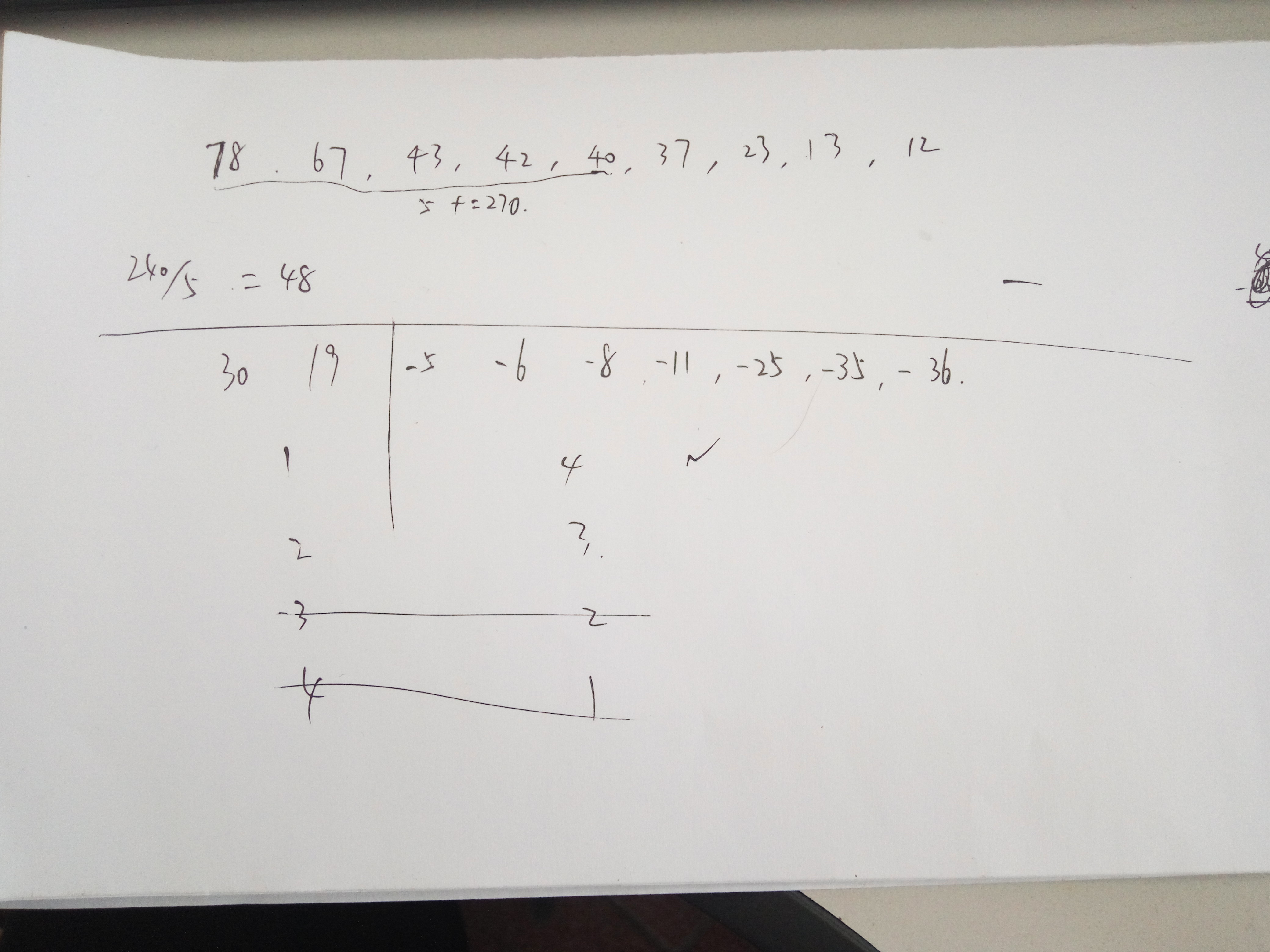Java数据结构算法问题，求最优解

`````` Map<String,Integer> map=new HashMap<String,Integer>();
map.put("tom",78);
map.put("jerry",42);
map.put("marry",12);
map.put("hugh",37);
map.put("aaron",23);
map.put("john",40);
map.put("white",43);
map.put("chris",13);
``````

1、第一优先的是人数，凑够钱买物品的人的组合里，人数最少的
2、第二优先的是价格，要求超过240，但是离240最接近的一组，因为从大到小排列一定能得到人数最少的，但是可能会比目标数额大很多，导致找零太多

10个回答

``````    public static List<Integer> limitValSubsequence(int[] sequence, int limitValue) {
List<Integer> retList = new ArrayList<>();

int[] sortSeq = Arrays.copyOf(sequence, sequence.length);
Arrays.sort(sortSeq);

int sumVal = 0;
int k = 0;
for (int i = sortSeq.length - 1; i >= 0; i--) {
sumVal += sortSeq[i];
if (sumVal > limitValue) {
k = sortSeq.length - i;
break;
}
}
if (k == 0) {
System.err.println("No subsequence math condition to > " + limitValue);
return retList;
}

limitValSubsequencePart(sortSeq, k, sequence.length - 1, limitValue, retList);
return retList;
}

public static int limitValSubsequencePart(int[] sequence, int count, int right, int limitValue,
List<Integer> outSeq)
{
outSeq.clear();
if (count <= 0) return Integer.MIN_VALUE;       //不可选了
if (right < 0) return Integer.MIN_VALUE;
int curVal = sequence[right];
if (limitValue > count * curVal) return Integer.MIN_VALUE;

if (right == 0) {
if (curVal > limitValue && count == 1) {
return curVal;
} else {
return Integer.MIN_VALUE;
}
}

//if curVal in
List<Integer> curInSeq = new ArrayList<>();
int inValue = Integer.MIN_VALUE;    //预设无效
if (curVal > limitValue && count == 1) {
inValue = curVal;
} else {
List<Integer> inSubSeq = new ArrayList<>();
int subVal = limitValSubsequencePart(sequence, count - 1, right-1, limitValue - curVal, inSubSeq);
if (subVal == Integer.MIN_VALUE) {//无效
inValue = Integer.MIN_VALUE;
} else {
inValue = curVal + subVal;
}
}

//if curVal not in
List<Integer> curOutSeq = new ArrayList<>();
int outValue = limitValSubsequencePart(sequence, count, right-1, limitValue, curOutSeq);

if (inValue == Integer.MIN_VALUE) {
return outValue;
} else if (outValue == Integer.MIN_VALUE) {
return inValue;
} else {
if (curInSeq.size() < curOutSeq.size()) {
return inValue;
} else if (curInSeq.size() > curOutSeq.size()) {
return outValue;
} else {
if (inValue > outValue) {
return outValue;
} else {
return inValue;
}
}
}
}

public static void testLimitValArray() {
final int NUMBER = 150;
int[] inputs = new int[NUMBER];
for (int i = 0; i < NUMBER; i++) inputs[i] = i+1;
int limitValue = NUMBER * 10;
long startTime = System.currentTimeMillis();
List<Integer> vals = limitValSubsequence(inputs,  limitValue);
int sumVal = 0;
for (int val : vals) sumVal += val;
long usedTime = System.currentTimeMillis() - startTime;
System.out.println("testLimitValArray limit:" + limitValue +  ", sum:" + sumVal + ", elems:" + vals + ", usedTime:" + usedTime);
}

``````

testLimitValArray limit:1500, sum:1501, elems:[46, 141, 142, 143, 144, 145, 146, 147, 148, 149, 150], usedTime:9565

1，把所有的value取出来，用9层for循环 for（int i =0 ,i < =1 ,i++） 判断结束条件为 sum = 78i+42j+12x+。。。。 > =240;
2, 把每种方式取出来，做比较先去人数最少的 就是变量0最多的一个。如果相等比较钱数最小的，

。大致的想法就这样，具体实现还得自己根据需求优化simuyinxi emmmm，这只是个例子，我们实际开发场景可能就不是9个对象了，可能动辄一两百个，总不能用一两百个循环吧.....这个要用到算法，好像是深度优先遍历什么的，要用递归的，我大概知道方向，但是还是不会解决
2 年多之前 回复2 年多之前 回复Aries_Jacky 不错
2 年多之前 回复2 年多之前 回复qq_40869023 嗯嗯
2 年多之前 回复simuyinxi 谢谢，你的思路是对的，我也在网上按照你的思路找到了相应的算法解答，后面有个大佬把代码给出来了，就只能把答案给他啦！还是谢谢你的帮助！
2 年多之前 回复daniel_miau 啊，是不是可以这样，你看，取到头五个数，然后头五个数相加是270比240大30，把30给减出来，用这五个数分别减去30，然后查一查后面的数有没有，如果有的话直接替换了就正好了，如果没有再减去29看有没有，以此类推，直到减至1如果还没有，那就是这五个数了
2 年多之前 回复

//获取最大个数方法就不写了。例子是3个数，思路是获取任意3个数据元素组合 结果存到map中 最后比较map结果，完全按照需求啊 求分

`````` int a[] = {60,55,50,45,40,30,20,15};
int target = 120 ;
//首先获取最大个数
int minNum = 3;
Map<String,Integer> map = new HashMap<String,Integer>();
//取出数组下任意元素组合下标
for(int i=0;i<=(a.length-minNum+1);i++){
for(int j=0;j<a.length;j++){
String flag = "";
int maxIndex = 0;
int total = 0;
for(int k=0;k<minNum;k++){
if((k+1) == minNum){
flag = flag + (k+j+i)+",";
maxIndex = k+j+i;
if(maxIndex >= a.length){
break;
}
total = total + a[(k+j+i)];
}else{
flag = flag + (k+i)+",";
total = total + a[(k+i)];
}
}
if(maxIndex >= a.length){
break;
}
System.out.println(flag + "======" + total);
map.put(flag, total);
}
}

//对map结果进行120匹配即可
``````a718089112 第二个for循环条件可以优化一下：int j=0;j<a.length-minNum;j++
2 年多之前 回复

1、基础数据的集合，即map，最好事先用TreeMap，因为容器内元素天然有序，对我们后面的计算会减少很多时间。
2、得到一个有序集合后，先从大到小取到满足条件的最少人数。
3、剩下的就是遍历，比较了；
ps：这种问题肯定避免不了遍历和递归这种操作，最优方案无非是在时间复杂度和空间复杂度上做权衡，如果是实际业务上的问题，再结合一些现实因素；

public void test4() {
Map map = new HashMap();
int[] values = {78,42,12,37,23,40,67,43,13};
Arrays.sort(values);
System.out.println(Arrays.toString(values));
int sum = 0;
for(int i = values.length-1;i>=0;i--) {
sum+=values[i];
map.put(keys[i], values[i]);
if(sum>=240) {
sum -= values[i];
break;
}
}
for (int i = 0; i < values.length; i++) {
if(sum+values[i]>=240) {
sum+=values[i];
map.put(keys[i], values[i]);
System.out.println(sum);
System.out.println(map);
break;
}
}
}